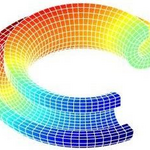Community Profile# Wan Ji

### Wuhan University

Last seen: 3 days ago Active since 2021

Research Interest: computational mechanics and modeling the world

Programming Languages:
Python, C++, C, MATLAB, Fortran
Spoken Languages:
English, French, Mandarin

#### Statistics

All
••••••••••••#### Content Feed

View by

Solved

The Piggy Bank Problem
Given a cylindrical piggy bank with radius g and height y, return the bank's volume. [ g is first input argument.] Bonus though...

1 year ago

Solved

Calculate cosine without cos(x)
Solve cos(x). The use of the function cos() and sin() is not allowed.

1 year ago

Solved

Approximate the cosine function
Without using MATLAB trigonometric functions, calculate the cosine of an argument |x| to a precision of |0.0001| *Hint:* You ...

1 year ago

Solved

Calculate a Damped Sinusoid
The equation of a damped sinusoid can be written as |y = A.&#8519;^(-&lambda;t)*cos(2πft)| where |A|, |&lambda;|, and |f| ...

1 year ago

Solved

Solve a System of Linear Equations
*Example*: If a system of linear equations in _x&#8321_ and _x&#8322_ is: 2 _x&#8321;_ + _x&#8322;_ = 2 _x&#8321;...

1 year ago

Solved

Verify Law of Large Numbers
If a large number of fair N-sided dice are rolled, the average of the simulated rolls is likely to be close to the mean of 1,2,....

1 year ago

Solved

Find the Oldest Person in a Room
Given two input vectors: * |name| - user last names * |age| - corresponding age of the person Return the name of the ol...

1 year ago

Solved

Convert from Fahrenheit to Celsius
Given an input vector |F| containing temperature values in Fahrenheit, return an output vector |C| that contains the values in C...

1 year ago

Solved

Calculate Amount of Cake Frosting
Given two input variables |r| and |h|, which stand for the radius and height of a cake, calculate the surface area of the cake y...

1 year ago

Solved

Times 2 - START HERE
Try out this test problem first. Given the variable x as your input, multiply it by two and put the result in y. Examples:...

1 year ago